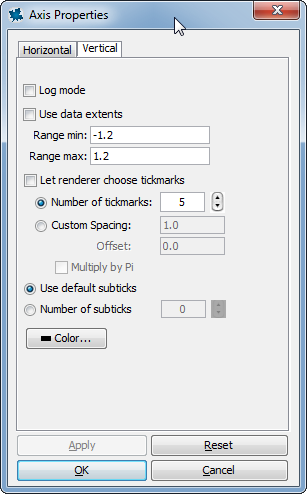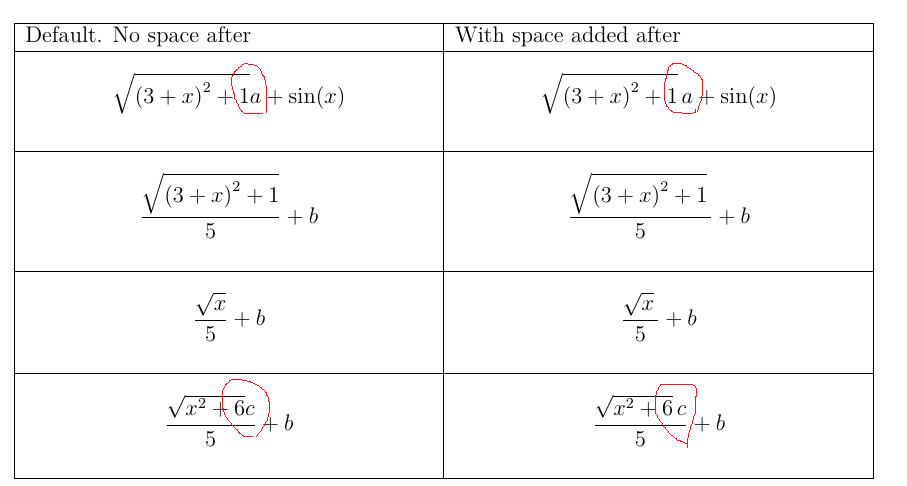## largest distance ...

Dear all

I have two matrices represent ( f(x[i],y[j])  and  g(x[i],y[j]) of a  given two dimensional functions.

How can I use thoses values of matrices to compute the largest distance between the two curves.

Many thanks

## Error, (in dsolve/numeric/process_input) system mu...

When i try to solve the problem i got error like this

 >>>(1)
 >(2)
 >(3)
 >(4)
 >(5)
 >(6)
 >>>## Disabling autoscaling when exploring a plot...

I am doing the first interactive plot in Tutorial 9:  Dynamic Applications.    The first part is Exploration Assistant.   This is mostly going OK but I don't want to have it rescale the y-axis to the data extents.   So I uncheck the box and type in my min & max values as shown below.    But when I drag the slider for coefficient a or b, the plot reverts back to using data extents.   How do I override this so that the axis range stays fixed when I drag the sliders?## Can I have an apostroph in cat ?...

I'd like to generate a string where the first part is changeable, and the second one needs to include apostrophs.

NODESteelProfiles_I:-Property("allmembers")

The section until til left bracket can be stored in one variable, but how can I add "allmembers") into the string?

## Use of add or sum commands with the LieAlgebras pa...

Hi,

The evalDG command included in the LieAlgebras via the DifferentialGeometry package allows summations such as evalDG(e1+e2) where e1 and e2 are two generators of a given Lie algebra.

Now, let L be a list of such summations, e.g.,

L= [e1+a*e3,2*e2+b*e4,e1+3*e5]

where a and b are symbols for variable names in the real domain.
Then, while the code

evalDG(add(eval(cat(e,i)),i=1..n));

works fine (n be a given integer), the code

evalDG(add(eval(L[i]),i=1..n));

does not, and an error message results due to an "invalid subscript selector".

What is the right code to realize this summation?

Thanks.

Jaqr

## How to get specific structure of the NullSpace ?...

Dear all,
I would like to get the Null Vectors of some matrix B but not those which are returned by NullSpace(B), i would like to get specific structure of the Kernel, i mean, i want to get the following structure of the Kernel when the matrix B is 10*10 for example:

Kernel= {
Vector[column](10, [0, 0, 0, 0, 0, 0, 0, 0, 0, alpha]),
Vector[column](10, [0, 0, 0, 0, 0, 0, 0, 0, alpha, beta]),
Vector[column](10, [0, 0, 0, 0, 0, 0, 0, alpha,beta, gamma]),
Vector[column](10, [0, 0, 0, 0, 0, 0, alpha, beta, gamma, delta])
}
Is it possible to do that with Maple ? Have you some ideas ?
In other words,  could you help me please ?

Best regards

## adding space after sqrt to improve the Latex?...

What do folks think about adding \, space automatically after Maple's Latex output for sqrt command?

This is all using the new Latex command. Not the orginal latex() command.

For me, it looks much better. I did some tests, and see no negative effect by always adding \, at the end of \sqrt{}

The reason is this: If there is a symbol after sqrt(.....)<HERE> then the math looks like the symbol is almost inside the integral, when it should be out. By adding \, this makes it more clear and better looking.

It is possible to write macro in Latex to do this in preamble I suppose. But if it can be done in the source, then that will be much better as it will always be there without the need to write complicated macro to change it later in Latex.

Here are 4 expressions to compareOne can see the current problem area and the effect of adding \, automatically. The space was added for each case above in the second column, even for those where it was needed (second and third expressions) to show that adding space has no negative side-effect even when not needed.

Here is the above raw latex used, compiled on Tex Live 2020 on Linux,  and the Maple worksheet below that.

\documentclass[12pt]{article}
\usepackage{amsmath}
\usepackage{longtable}
\usepackage{mleftright}
\mleftright

\begin{document}

\begin{longtable}{|p{3in}|p{3in}|}\hline
Default. No space after & With space added after\\\hline
$\sqrt{\left(3+x \right)^{2}+1} a +\sin \left(x \right)$
&
$\sqrt{\left(3+x \right)^{2}+1}\, a +\sin \left(x \right)$\\\hline
$\frac{\sqrt{\left(3+x \right)^{2}+1}}{5}+b$
&
$\frac{\sqrt{\left(3+x \right)^{2}+1}\,}{5}+b$\\\hline
$\frac{\sqrt{x}}{5}+b$
&
$\frac{\sqrt{x}\,}{5}+b$
\\\hline
$\frac{\sqrt{x^{2}+6} c}{5}+b$
&
$\frac{\sqrt{x^{2}+6}\, c}{5}+b$\\\hline
\end{longtable}

\end{document}

 > interface(version); Physics:-Version();> restart;
 > Latex:-Settings(UseImaginaryUnit=i,       UseColor = false,       powersoftrigonometricfunctions= computernotation,       leavespaceafterfunctionname = true,       cacheresults = false );> expr1:=sqrt((3+x)^2+1)*a+sin(x); Latex(expr1)\sqrt{\left(3+x \right)^{2}+1} a +\sin \left(x \right)

 > expr2:=sqrt((3+x)^2+1)/5+b; Latex(expr2)\frac{\sqrt{\left(3+x \right)^{2}+1}}{5}+b

 > expr3:=sqrt(x)/5+b; Latex(expr3)\frac{\sqrt{x}}{5}+b

 > expr4:=(sqrt((3+x^2)+3)*c)/5+b; Latex(expr4)\frac{\sqrt{x^{2}+6} c}{5}+b

 >

(typo, should be sqrt, not int)

## why typing something again, makes it integrate?...

I am having hard time understanding why Maple does this.

I have an integral, which maple could not integrate. So it returns int(....,x). Which is all fine. Then I used subsindets to force all terms in form e^(ln()+ln()+...) to expand in order to simplify the integrand, just for display purposes.

subsindets returns back the integral unevaluated (as expected) but with integrand a little simpler, again as expected.

All is well so far.

Then I find to my surprise, if I type the result back one more type, now the the integral actually evaluates.

Why?

I have 2 questions on this.

1) If the original integral did not evaluate, why simplifying e^(ln()+ln()+...) makes it now evaluate? Did not Maple know this allready?

2) Why result back from subsindets remained unevaluated integral, and I had to type it again to see it now evaluates?

I found this after long time debugging, since this was done in code, not looking at screen.

What happened is this: I store the result  of subsindets in a variable, and when I look at it in the debugger, I see int() still there, as expected.

I call a function to return back this result. I now see int() is gone!   So the act of just returning the result back, caused it to evaluate. Even though the orginal variable still had int() in it as. So returning the expression back, was equivalent to typing it again on the screen in the example below, which caused it to evaluate.

Please see worksheet below.

 > interface(version);> Physics:-Version();> restart;
 > expr:=int(-1/2*exp((ln(a+cos(1/2*x)^2)+(-2*a-2)*ln(cos(1/2*x)))/a)*(-1+cos(x))/sin(1/2*x)/cos(1/2*x)/(a+cos(1/2*x)^2),x);> #try again expr;> expr:=subsindets(expr,'specfunc( anything, exp )',f->(if(has(op(1,f),'ln'),expand(f),f)));> #notice, the above is still int. Why is typing expr again, now makes it evaluate?? expr>

Another simpler example, is just doing assignment to new variable. This causes evaluation.

restart;
expr:=int(-1/2*exp((ln(a+cos(1/2*x)^2)+(-2*a-2)*ln(cos(1/2*x)))/a)*(-1+cos(x))/sin(1/2*x)/cos(1/2*x)/(a+cos(1/2*x)^2),x);
expr:=subsindets(expr,'specfunc( anything, exp )',f->(if(has(op(1,f),'ln'),expand(f),f)));
#the above still has int() in it.

#this assignment, also causes evaluation
A:=expr;

## converts a Maple polynomial into a list of its coe...

converts a Maple polynomial into a list of its coefficients.

## How to find integration of max of two functions?...

Suppose we have a parametric function g(x)=max{h(x,p),f(x,p)}. How can I find the exact form of g(x) by conditioning on x and also how I can find the integration of g(x) over an interval [a,b]?

For example, g(x)= max{ x-p, x^2+px} and [a,b]=[0,10].

## Numerical Solution for system of Differential Equa...

I want to solve the following system of differential equations to find xphi, but I seem to be doing something wrong because I get an error message.

xodephi := {diff(x(t), t) = 16250.25391*(1 - (487*x(t))/168 + 4*Pi*x(t)^(3/2) + (274229*x(t)^2)/72576 - (254*Pi*x(t)^(5/2))/21 + (119.6109573 - (856*ln(16*x(t)))/105)*x(t)^3 + (30295*Pi*x(t)^(7/2))/1728 + (7.617741607 - 23.53000000*ln(x(t)))*x(t)^4 + (535.2001594 - 102.446*ln(x(t)))*x(t)^(9/2) + (413.8828821 + 323.5521650*ln(x(t)))*x(t)^5 + (1533.899179 - 390.2690000*ln(x(t)))*x(t)^(11/2) + (2082.250556 + 423.6762500*ln(x(t)) + 33.2307*ln(x(t)^2))*x(t)^6)*x(t)^5, diff(xphi(t), t) = 5078.204347*x(t)^(3/2), x(0) = 0.03369973351, xphi(10.92469316) = 0}

xsolphi := dsolve(xodephi, numeric)

Error, (in dsolve/numeric/bvp) precision is insufficient for required absolute error, suggest increasing Digits to approximately 25 for this problem

Later, I am interested in plotting xphi for t ranging from (11.92469316 - 1) to 11.92469316, but first I need to obtain a numerical solution for xphi in this region. Any advice?

## corrupted worksheet...

Hey

Can anyone help me with this file?

I don't know how it happend, but the file somehow got corrupted. Is there anything i can do?

Thanks

Videregående_beton_Gang_9.mw

## Why does Matlab CodeGeneration fail to optimize, a...

A := Matrix(2, 2):
CodeGeneration:-Matlab(unapply(A), optimize, resultname = "A");

Warning, unable to optimize
Warning, procedure/module options ignored
function Areturn = A()
Areturn = [0 0; 0 0;];

What is this behaviour. I can't find any explanation online.

## Can Maple represent 2D density plots?...

Suppose you do this

restart:
with(plots):
with(Statistics):
A := Sample(RandomVariable(Normal(0, 1)), 10^4):
B := Sample(RandomVariable(Normal(0, 1)), 10^4):
ScatterPlot(A, B, symbol=point);


The plot shows a greater density of dots around (0, 0) and they become increasingly sparse as one moves away from (0, 0).
A pretty way to represent such clouds is to do "2D density plots".
You will find some example from R here  2d-density-plot-with-ggplot2.html

For the moment I do something like this (which is to be adpated for almost any particular case).
My main question is: do recent versions of MAPLE possess something similar to R's features?

 > restart:
 > interface(version);(1)
 > with(Statistics):
 > UseHardwareFloats := false: N := 10^5: A := Sample(RandomVariable(BetaDistribution(2, 6)), N): B := Sample(RandomVariable(BetaDistribution(6, 2)), N): nb := 50: mA := min(A): hA := Range(A)/(nb-1): mB := min(B): hB := Range(B)/(nb-1): cA := floor~((A-~mA)/~hA)+~1: cB := floor~((B-~mB)/~hB)+~1: NP := Matrix(nb\$2, 0): for n from 1 to N do   NP[cA[n], cB[n]] := NP[cA[n], cB[n]]+1 end do: NP:
 > plots:-matrixplot(   NP,   heights=histogram,   colorscheme=["Blue", "White", "Red"],   orientation=[270, 0, 0],   style=patchnogrid,   lightmodel=none,   labels=["A", "B", ""],   axis=[tickmarks=[nb+1=max(B), 1=mB]],   axis=[tickmarks=[nb+1=max(A), 1=mA]] )>